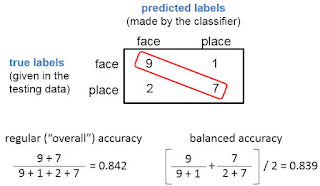## Friday, December 18, 2015

### balanced accuracy: what and why?

I was a bit thrown at OHBM by mentions of using "balanced" accuracy to describe classification performance ... what's wrong with "regular" accuracy? Well, nothing is wrong with "regular" accuracy, if your dataset is already balanced (meaning equal numbers of trials in each class and cross-validation fold, which I very highly recommend). But if your test set is not balanced, "balanced" accuracy is probably better than "regular".At left is an example of a confusion matrix produced by a classifier, where the test set was balanced, with 10 examples of class face, and 10 of class place. The classifier did quite well: 9 of the 10 face examples were (correctly) labeled face, and 8 of the 10 place examples were (correctly) labeled place. For lack of a better term, what I'll call "regular" or "overall" accuracy is calculated as shown at left: the proportion of examples correctly classified, counting all four cells in the confusion matrix. Balanced accuracy is calculated as the average of the proportion corrects of each class individually. In this example, both the overall and balanced calculations produce the same accuracy (0.85), as will always happen when the test set has the same number of examples in each class.This second example is the same as the first, but I took out one of the place test examples: now the testing dataset was not balanced, as it has 10 face examples, but only 9 place examples. As you can see, now the overall accuracy is a bit higher than the balanced.

It's easy to generate more confusion matrices to get a feel for how the two ways of calculating accuracy differ, such as with this R code:

`````` c.matrix <- rbind(c(1,0),
c(1,1));
sum(diag(c.matrix))/sum(c.matrix);  # "overall" proportion correct
first.row <- c.matrix[1,1] / (c.matrix[1,1] + c.matrix[1,2])
second.row <- c.matrix[2,2] / (c.matrix[2,1] + c.matrix[2,2])
(first.row + second.row)/2; # "balanced" proportion correct
``````

So, should we use balanced accuracy instead of overall? Yes, it's probably better to use balanced accuracy when there's just one test set, and it isn't balanced. I tend to be extremely skeptical about interpreting classification results when the training set is not balanced, and would want to investigate a lot more before deciding that balanced accuracy reliably compensates for unbalanced training sets. However, it's probably fine to use balanced accuracy with unbalanced test sets in situations like cross-classification, where a classifier is trained once on a balanced training set (e.g., one person's dataset), and then tested once (e.g., another person's dataset). Datasets requiring cross-validation need to be fully balanced, because the each testing set contributes to the training set in other folds.

For more, see Brodersen, Kay H., Cheng Soon Ong, Klaas E. Stephan, and Joachim M. Buhmann. 2010. "The balanced accuracy and its posterior distribution." DOI: 10.1109/ICPR.2010.764